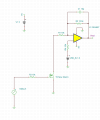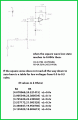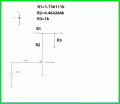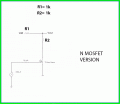# Non Inverting Summing Amplifier Design

#### hoyyoth

Joined Mar 21, 2020
253
Dear Team,

I need to design a summing amplifier with 2 inputs.

My input one is a DC voltage source of value 1.6V and the second one is a square wave whose amplitude is 5V and max frequency is 1Mhz.

The requirement is when the square wave is LOW(0V) the summer output should be 1.6V and when the square wave is high(5V) the summer output

should be 3.6V.

May I know how to approach this?

Regards
HARI

#### crutschow

Joined Mar 14, 2008
32,062
Sounds like homework.
Do you know the configuration of a summing amp?
If so, show it and how you think the values resistor values would be calculated.

#### DickCappels

Joined Aug 21, 2008
9,872

#### hoyyoth

Joined Mar 21, 2020
253
Sounds like homework.
Do you know the configuration of a summing amp?
If so, show it and how you think the values resistor values would be calculated.
Hi ,
It is not a home workI implemented the circuit as shown below.I am thinking of doing it in a simple way.

#### crutschow

Joined Mar 14, 2008
32,062
For your problem you don't necessarily need a summing amp, you can just use the correct value resistors to change the square-wave voltage.

Edit: On second thought, I don't think it can be done with resistors only.

Last edited:
•Delta Prime

#### MrAl

Joined Jun 17, 2014
10,087
Dear Team,

I need to design a summing amplifier with 2 inputs.

My input one is a DC voltage source of value 1.6V and the second one is a square wave whose amplitude is 5V and max frequency is 1Mhz.

The requirement is when the square wave is LOW(0V) the summer output should be 1.6V and when the square wave is high(5V) the summer output

should be 3.6V.

May I know how to approach this?

Regards
HARI
Hello there,

When you use a summer you normally use the inverting input as the summing node because that makes it simpler with two resistors for the two inputs and one feedback resistor. The resistor values set the summing factors and the feedback resistor sets the overall gain.
There is just one little catch: the output will be inverted. That is, it will be the negative of the actual sum. So if you sum 1v + 2v with a gain of 1 the output will not be 3v it will be -3v. This means it doesnt really work unless you can put up with a negative output (or positive when you want negative).

To remedy this, you can use smack another op amp on the output to invert the output to get it to go positive as you need, but then that requires another op amp section.
Another idea is to just design a non inverting adding circuit that acts like a true summer but does not invert the output. This does require an extra resistor though in addition to the three plus the one op amp section.

To figure out the resistor values, you do a circuit analysis of a non inverting op amp circuit but place two resistors at the non inverting input rather than the inverting input,. The addition comes from the addition of voltages we see when we use two resistors in series and two different voltage sources going to the two ends of the two resistors and the center junction becomes the addition node. The voltages add in a slightly more unusual way but the do still add. The 'unusualness' of the addition is made up by adjusting the gain of the op amp, knowing the overall gain is the feedback resistor divided by the resistor going to ground from the inverting input plus 1. This is often stated like so:
G=Rf/Rin+1

Fairly simple. The next thing is to calculate the voltage that results at the non inverting terminal, then multiply by G. The result looks like this:
Vout=(G+1)*((v1*R2)/(R2+R1)+(v2*R1)/(R2+R1))
(although there G is really Gm1 which is G-1)

where v1 would be the 'offset' voltage 1.6v and v2 would be the square wave that you said goes from 0 to 5v for an output of 1.6 to 3.6 volts. The gain is as above.

To calculate the resistor R2 and gain G you can form two equations one with v2=0 and one with v2=5 and both with v1=1.6, and the one with v2=0 is equated to 1.6 and the other is equated to 3.6 and that gives you two equations in two unknowns R2 and G. You then solve for R2 and G simultaneously. R1 is selected arbitrarily or based on the required input impedance, so R1 can be 1k, 2k, 10k, or anything in between or even higher. In the example below i used R1=1k.

As a side note, since the offset is 1.6v and the range of outputs is always either 1.6 or greater, there may be a solution with just two resistors. The problem with an exact solution though is i think one or more resistor values would come out negative which doesnt work unless you want to construct a negative resistor (which is not simple anyway) but there may be an approximate solution. Because the solution above results in an overall gain of greater than 1 (which was 1.4) i dont think it will work for this particular application.

The solutions are as follows and the circuit is such that:
R1 is connected to the constant DC source (and to the non inverting input of the op amp),
R2 is connected to the square wave (and to the non inverting input of the op amp),
Rf is the feedback resistor (connected output to inverting input),
Rin is the resistor from the inverting input to ground,
v1 is the constant DC voltage source voltage (referenced to ground),
v2H is the square wave highest voltage (referenced to ground, 5v in this thread),
v2L is the square wave lowest voltage (assumed to be 0v in these solutions),
voH is the highest output voltage, (3.6v in this thread),
voL is the lowest output voltage (1.6v in this thread),
Gm1 is the gain minus 1 which is more convenient than calculating G, and Gm1=G-1.

Formulas:
Gm1=G-1=(v2H*voL-v1*voL+v1*voH-2*v1*v2H)/(v1*v2H)
R2=(R1*v2H*voL)/(v1*voH-v1*voL)
Rf=Rin*Gm1

To use the formulas, calculate Gm1, then choose R1 based on your input impedance requirements, then calculate R2, then choose Rin to be some reasonable value, then calculate Rf

For an example, with:
v1=1.6v
v2H=5v
v2L=0v (an assumption throughout this discussion)
voL=1.6v
voH=3.6v

and choosing:
R1=1k
Rin=10k

we get:
Gm1=2/5
R2=2.5k
Rf=4k

You can check these values with a simulator.
I used the assumption that v2L is 0v (the square wave low voltage level) because that simplifies the solutions. Without that we end up with:
Gm1=-((v2H-v1)*voL+(v1-v2L)*voH+v1*v2L-v1*v2H)/(v1*v2L-v1*v2H)
R2=-(R1*v2H*voL-R1*v2L*voH)/(v1*voL-v1*voH)
and always check that the solutions are valid and reasonable.

One final little note...
It is tempting to form three equations, one with the square wave at 0 and the second with the square wave at 5v, and a third one with the square wave at the average of 0v and 5v and the output as the average output (valid because we expect a perfect linear response), then solve for all three variables: G, R1, and R2. The problem here though is that R1 is arbitrary so there is no unique solution, and also i think precalculating a new equation like that does not introduce a new linearly independent equation so that idea fails to add any new information to the equation set.

Last edited:
•hoyyoth

#### MrAl

Joined Jun 17, 2014
10,087
Hello again,

Taking another look at your circuit i can see you are using a MOSFET to generate the square wave and that would require a pullup resistor for the solution i provided in the last post. The pullup resistor changes the circuit slightly so the values have to change slightly to get near perfect results. Without the alterations in values the high output would be around 3.56 volts instead of 3.60 volts, but since we are doing it why not throw everything we've got at it. The equations come out quite a bit more complicated though so i dont present them here just the solutions. The main calculations are to calculate the gain and the resistor R5 (see attachment) as everything else is either arbitrary or somewhat arbitrary. For example, R4 is chosen to be 10k while R6 (the pullup resistor) is chosen to be 1k.

In the attachment we see the circuit modified and also a pullup resistor added (R6). The other values were calculated previously and they are shown on the schematic next to each part. Because of the added pullup resistor those values result in that slight error, so the circuit was analyzed again and new values were calculated and these are shown below the schematic. Below that there is a complete table in case the MOSFET can not pull the voltage at the right side of R6 exactly to ground. The values change slightly for offsets that could cause so the table matches 5 possible offset voltages to new values for R2 and R5.
The change is roughly 95 percent per 0.1 volt offset, but the table is more accurate.
If it turns out you have an intermediate value like 0.15v offset, then you could extrapolate between the values for 0.1v and those for 0.2v, and that should be close enough.
If you like i can post the formulas but there are quite a few characters in the formulas for R2 and R5 so you may not want to be bothered with that (there are 164 characters in the solutions for Gm1 and R5 and that is with only the square wave low state voltage being varied, the full solution is easily over 2000 characters).

As a final note, in the previous post the solution had shown a feedback resistor value of 5k but really that was supposed to be 4k, and so the post was corrected.Last edited:

#### AnalogKid

Joined Aug 1, 2013
10,308
If you don't want to mess with a summing circuit, you can do this with an analog multiplexer, two trimpots, and a voltage follower output buffer. No gains, no offsets, no PIC or Arduino, and no 555.

If the system +5 V supply is not stable enough for your output requirements, you have to add a voltage reference such as an LM4040. But that also is true of the summing approach.

OR - No multiplexer. A 2-resistor divider uses the +5 V to create +3.6 V. A third resistor is switched in parallel with the lower resistor to GND, using a MOSFET as in post #4, to create +1.6 V. As above, the output goes through a unity-gain buffer.

There is another way with even fewer parts, but it needs data.

What is the source of the controlling square wave? What kind of load can it drive?

ak

•hoyyoth

#### MrAl

Joined Jun 17, 2014
10,087
Hello again,

Actually because there is a transistor there is a very simple solution if the output impedance can be above zero (like 1k or something). See attachment.
The values can be adjusted for a different output impedance quite easily the formulas are very simple for this circuit. In this way we can even drop the 1.6v reference.
Just in case the output has to be inverted from what this circuit does, we could use a P MOSFET instead of an N MOSFET device.
Vout is at the top of R3.•hoyyoth

#### hoyyoth

Joined Mar 21, 2020
253
If you don't want to mess with a summing circuit, you can do this with an analog multiplexer, two trimpots, and a voltage follower output buffer. No gains, no offsets, no PIC or Arduino, and no 555.

If the system +5 V supply is not stable enough for your output requirements, you have to add a voltage reference such as an LM4040. But that also is true of the summing approach.

OR - No multiplexer. A 2-resistor divider uses the +5 V to create +3.6 V. A third resistor is switched in parallel with the lower resistor to GND, using a MOSFET as in post #4, to create +1.6 V. As above, the output goes through a unity-gain buffer.

There is another way with even fewer parts, but it needs data.

What is the source of the controlling square wave? What kind of load can it drive?

ak
There is a small change in spec'
Let me explain my application.
I have a CLK with amplitude 5V and frequency 1Mhz is coming from an Arduino Board. I have DC source with it 's voltage(Let it be VDD) varies from 1.6V to 3.6V.
My circuit need to perform vary this Voltage in such a way that when CLK is Low circuit need to provide an output of VDD/2 and when CLK is high the circuit needs to provide an output of VDD.
Let me take an example Let VDD=2V when the CLK I/P is Low my circuit output should be at 1V when CLK becomes high the circuit output should be at 2V.

Last edited:

#### hoyyoth

Joined Mar 21, 2020
253
Hello again,

Actually because there is a transistor there is a very simple solution if the output impedance can be above zero (like 1k or something). See attachment.
The values can be adjusted for a different output impedance quite easily the formulas are very simple for this circuit. In this way we can even drop the 1.6v reference.
Just in case the output has to be inverted from what this circuit does, we could use a P MOSFET instead of an N MOSFET device.
Vout is at the top of R3.

View attachment 239721
Thank you
Let me explain my application.

I have a CLK with amplitude 5V and frequency 1Mhz is coming from an Arduino Board. I have a DC source with its voltage(Let it be VDD) that varies from 1.6V to 3.6V.

My circuit needs to perform vary this Voltage in such a way that when CLK is Low circuit need to provide an output of VDD/2 and when CLK is high the circuit needs to provide an output of VDD.

Let me take an example Let VDD=2V when the CLK I/P is Low my circuit output should be at 1V when CLK becomes high the circuit output should be at 2V.

#### MrAl

Joined Jun 17, 2014
10,087
Thank you
Let me explain my application.

I have a CLK with amplitude 5V and frequency 1Mhz is coming from an Arduino Board. I have a DC source with its voltage(Let it be VDD) that varies from 1.6V to 3.6V.

My circuit needs to perform vary this Voltage in such a way that when CLK is Low circuit need to provide an output of VDD/2 and when CLK is high the circuit needs to provide an output of VDD.

Let me take an example Let VDD=2V when the CLK I/P is Low my circuit output should be at 1V when CLK becomes high the circuit output should be at 2V.
Hello again,

Ok that is a little different, but may make it even simpler yet. One question remains however, and that is what sort of output impedance would be acceptable. For example, would 500 Ohms be ok, or does it have to be lower like 100 Ohms, or even lower yet? Also, is it ok if the output impedance varies when the clock is low and when high.

If the output impedance can be 500 Ohms to 1000 Ohms then the simplest circuit i think then would be to use the transistor as before. Connect two 1k resistors in series and connect one loose end to Vdd and the other loose end to the drain of the MOSFET, then take the output from the junction of the two resistors.
If you use an N MOSFET you will get inversion with the clock, as long as the MOSFET turns on with very low resistance. Using a P MOSFET may also work with no inversion.

The circuit is shown in the attachment. This assumes you want to use a MOSFET too but it can be done with an op amp intead of a MOSFET if you rather do that.Last edited:
•hoyyoth

#### hoyyoth

Joined Mar 21, 2020
253
Hi Ai,

Thank you very much. The output will be connecting to the Gate of a MOS.

May I know how to implement this circuit using OPAMP. I prefer OPAMP one.

I tried some opamp circuits but when the I/P frequency becomes 1Mhz the output is not proper.

Regards
HARI

#### AnalogKid

Joined Aug 1, 2013
10,308
The circuit in #12 is almost there, but the logic polarity is reversed.

ak

•hoyyoth

#### hoyyoth

Joined Mar 21, 2020
253
The circuit in #12 is almost there, but the logic polarity is reversed.

ak
Hi Analog,
Opamp based solution #4 will be good I think.But for 1Mhz it is not working for me .I chaged OPAMP(used opamp with high slew rate ) and also used high speed mosftes but no luckRegards
HARI

#### ericgibbs

Joined Jan 29, 2010
17,431
hi hoy,
What impedance is the Vout driving into.?
E

#### hoyyoth

Joined Mar 21, 2020
253
hi hoy,
What impedance is the Vout driving into.?
E
Hi Eric,

It is driving the gate of MOSFET.

Regards
HARI

#### ericgibbs

Joined Jan 29, 2010
17,431
hi,
This would be my simple option.
E

#### Attachments

•crutschow

#### hoyyoth

Joined Mar 21, 2020
253
hi,
This would be my simple option.
E
Hi Eric,

Thank you.
May I know the role of diodes D1 and D2.Are they used for increasing the switching speed

Regards
AHRI